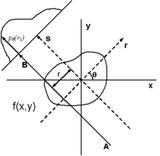Tomographic Reconstruction

Tomographic reconstruction

to get instant updates about 'Tomographic Reconstruction' on your MyPage. Meet other similar minded people. Its Free!

X

Description:
The mathematical basis for tomographic imaging was laid down by Johann Radon. It is applied in computed tomography to obtain cross-sectional images of patients. This article applies in general to tomographic reconstruction for all kinds of tomography, but some of the terms and physical descriptions refer directly to X-ray computed tomography.

The projection of an object at a given angle [itex]theta[/itex] is made up of a set of line integrals. In X-ray CT, the line integral represents the total attenuation of the beam of x-rays as ittravels in a straight line through the object. As mentioned above, the resulting image is a 2D (or 3D) model of the attenuation coefficient. That is, we wish to find the image [itex]mu(x,y)[/itex]. The simplest and easiest way to visualise method of scanning is the system of parallel projection, as used in the first scanners. For this discussion we consider the data to be collected as a series of parallel rays, at position [itex]r[/itex], across a projection at angle [itex]theta[/itex]. This is repeated for various angles. Attenuation occurs exponentially in tissue:

[itex]I = I_0expleft(right)[/itex]

where [itex]mu(x)[/itex] is the attenuation coefficient at position [itex]x[/itex] along the ray path. Therefore generally the total attenuation [itex]p[/itex] of a ray at position [itex]r[/itex], on the projection at angle...

No feeds found

AllPosting your question. Please wait!...